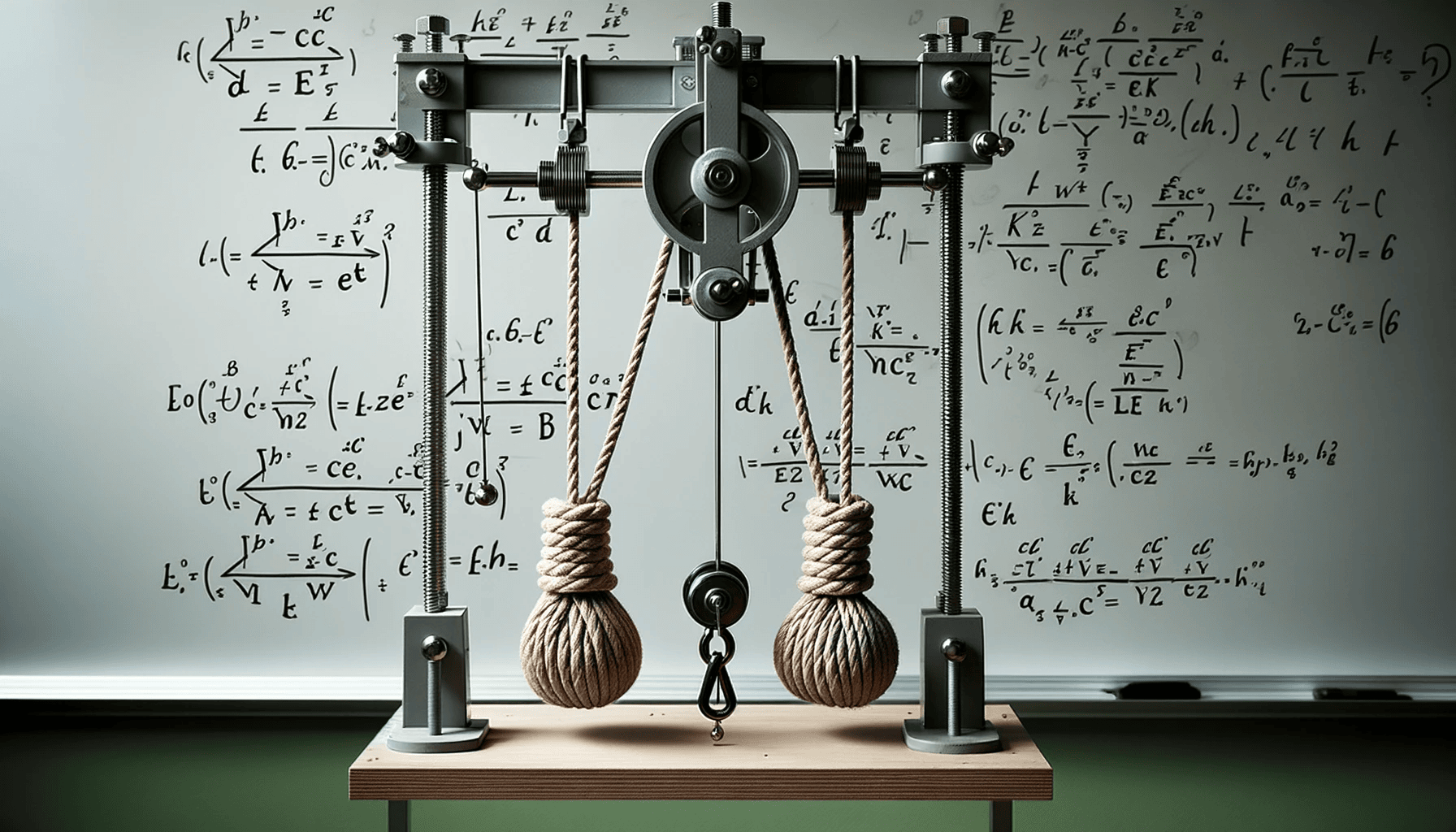# Unit 2.7 | Problem Solving – Simple Pulley Systems###### Jason Kuma

Writer | Coach | USC - Physics B.S & Business B.A. | Fremont, CA

###### Article Content
Simple pulleys, also called Atwood machine are a common force problem you’ll see. We’ll continue using the framework from before to solve pulley problems.

This course article contains videos that can only be accessed once enrolled in the Learn AP Physics from Scratch Course.

### Unit 2 Breakdown

You are on Lesson 7 of 8:

#### In this lesson you will learn:

• Understanding simple pulley systems (called an Atwood Machine)
• Solving pulley problems (the long way)
• Solving pulley problems (the easy way)

### The basics

The most basic pulley system, also referred to as an Atwood’s machine, consists of 2 masses connected by a rope. The tension is the same throughout the entire rope. The rope goes around a massless pulley as shown below.

And what if the pulley had mass? Then the mass would create a force called “Torque” which we will cover much later in Unit 6.

#### The direction of acceleration

The pulley system will always accelerate in the direction of the heavier mass.

### Solving Pulley Problems

There are two ways we can solve pulley problems.

1. Using our force frame worked covered in previous sections (the long way)
2. Solving as a system (the easy way)

I will explain how to do method one in the video below and cover method two in the next lesson (Lesson 2.8)

[Video for course members only]

### PQ – Pulleys (Intermediate)

1. Two objects (49.0 and 24.0 kg) are connected by a massless string that passes over a massless, frictionless pulley. The pulley hangs from the ceiling. Find the acceleration of the objects and the tension in the string.
2. A window washer pulls herself upward using the bucket–pulley system. She sits in the bucket, and when she pulls down on the rope the bucket moves up.
• How hard must she pull downward to raise herself slowly at constant speed?
• If she increases this force by 15%, what will her acceleration be? The mass of the person plus the bucket is 72 kg.

1. Block A (mA) of mass 13kg is held in place on a frictionless table. It is connected by a massless rope to Block B (mB) of mass 5kg, that is hanging off the table.
• Find the acceleration of each block when the system is released from rest.
• Now if Block A is released from rest 1.25m from the edge of the table. How long does it take to get there?
2. Let’s replicate the situation above. This time, however, the table has a coefficient of static friction of .4 (µs = .4) and a kinetic friction of .2 (µk = .4).
• What minimum value of mA will keep the system from starting to move?
• What value of ma will keep the system moving at constant speed?
1. A block of mass m1 (5kg) is on a ramp (µk = .22) that is inclined at 20° above the horizontal. It is connected by a massless string to a block of mass m2 (8kg) that hangs over the top edge of the ramp.
• What is the acceleration of the masses and the tension in the string?
• If m1 = 8 kg, and the coefficient of static friction between m1 and the incline is 0.300, find the maximum value of m2 that will not make m1 slide up the incline.

### Lesson 2.7 Recap

In this lesson you learned how to solve pulley problems in two different way.

### Lesson 2.8 Preview

In the next lesson, we will take “solving problems as a system” to the next step. We will cover how to solve problems where there are 2 or more objects — similar to what we did with the pulley system.###### Jason Kuma

Writer | Coach | USC - Physics B.S & Business B.A. | Fremont, CA

## Units in AP Physics 1

Unit 1 – Linear Kinematics

Unit 2 – Linear Forces

Unit 3 – Circular Motion

Unit 4 – Energy

Unit 5 – Momentum

Unit 6 – Torque

Unit 7 – Oscillations

# How to Solve All Difficult Force Problems – Framework

## Prepare for High School Physics## 3LqKuK5a5wuKEfphNdQ5wDJPKd1pzh1TEh## 3LqK...1TEh

1. Buy BTC on any platform. Strike, Cash App, and Venmo are the easiest to use.

2. Copy and paste the address above (or scan the QR) on the app.

3. Select a amount send.